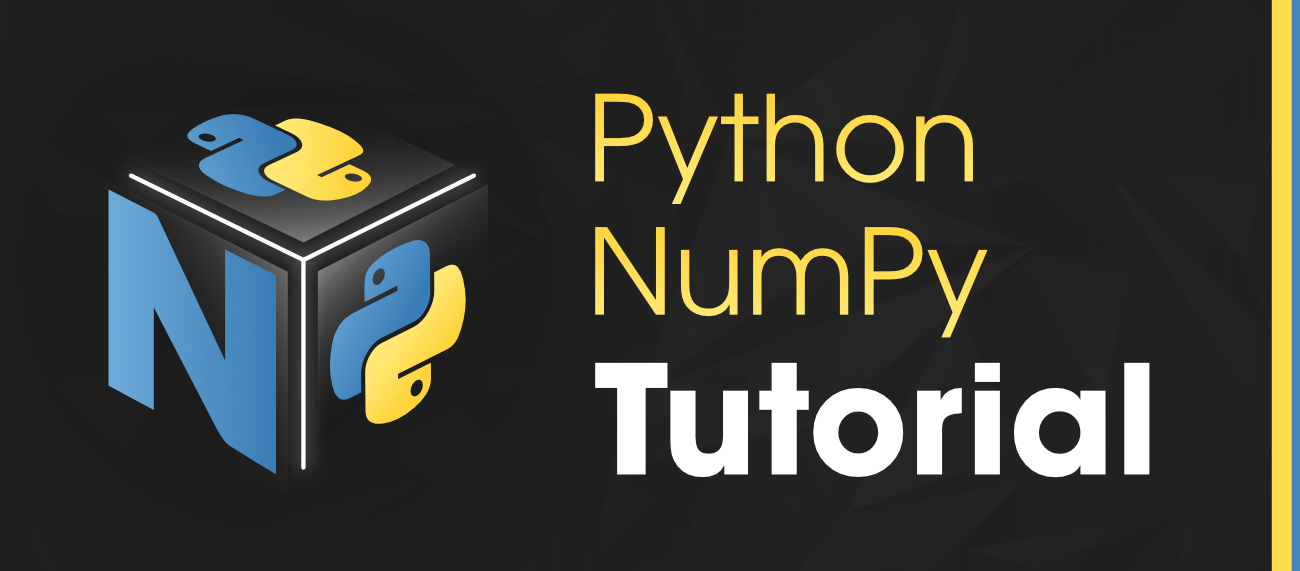Open In App
Related Articles
• Write an Interview Experience
• NumPy Tutorial

# NumPy Tutorial

Python NumPy is a general-purpose array processing package which provides tools for handling the n-dimensional arrays. It provides various computing tools such as comprehensive mathematical functions, linear algebra routines. NumPy provides both the flexibility of Python and the speed of well-optimized compiled C code. It’s easy to use syntax makes it highly accessible and productive for programmers from any background.

This NumPy tutorial helps you learn the fundamentals of NumPy from Basics to Advance, like operations on NumPy array, matrices using a huge dataset of NumPy – programs and projects.Recent Articles on NumPy !!